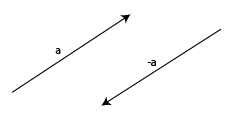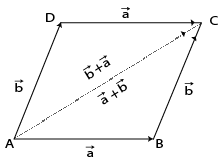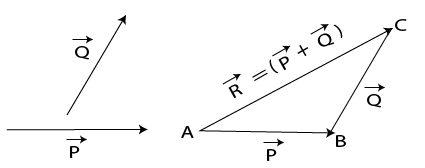Distributive vector multiplication

## Objective

We are going to verify the vector property. c⃗ x (a⃗+b⃗)= I c⃗ x a⃗ I + I c⃗ x b⃗ I.

## Pre-requisite Knowledge

• What are Vectors?
• Formula of area of a parallelogram
• Parallelogram Law of Addition of Vectors:
• Triangle Law of Addition of Vectors:

## What are Vectors?

A vector is a Latin word that means carrier. Vectors carry information from point A to point B. The length of the line between the two points A and B is called the magnitude of the vector, and the direction of the The displacement of point A from point B is called the direction of the vector AB. Vectors are also called Euclidean vectors or spatial vectors. Vectors have many applications in math, physics, computer science, engineering, and various other fields.

## Formula of area of a parallelogram

The area of a parallelogram is the base times the height. The area of a parallelogram is A = b x h.vector a

## Parallelogram Law of Addition of Vectors:

The law states that if two sides of a triangle AB & BC with P and Q as magnitudes represent the two vectors acting simultaneously on a body in the same order, then the third side AC WITH R as magnitude of the triangle represents the resultant vector R = P + Q.

###Parallelogram ABCD

## Triangle Law of Addition of Vectors:

The law states that if two co-initial vectors A and B act simultaneously, represented by the two adjacent sides AB & AD of a parallelogram ABCD, then the diagonal of the parallelogram AC represents the sum of the two vectors A and B. That is, the resultant vector starts from the same initial point.Triangle Law